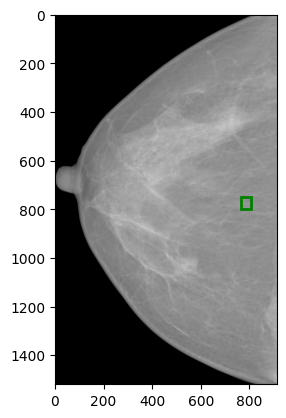# Adjust the bounding box based on ROI of the image

I have an original image of size (3518, 2800) as shown in the uploaded figure original_image.png. Now i have cropped the breast from the image and resized it to (1520, 912) as shown in the uploaded figure cropped_image.png. Following is the code for this preprocessing:

``````def np_CountUpContinuingOnes(b_arr):
# indice continuing zeros from left side.
# ex: [0,1,1,0,1,0,0,1,1,1,0] -> [0,0,0,3,3,5,6,6,6,6,10]
left = np.arange(len(b_arr))
left[b_arr > 0] = 0
left = np.maximum.accumulate(left)
# from right side.
# ex: [0,1,1,0,1,0,0,1,1,1,0] -> [0,3,3,3,5,5,6,10,10,10,10]
rev_arr = b_arr[::-1]
right = np.arange(len(rev_arr))
right[rev_arr > 0] = 0
right = np.maximum.accumulate(right)
right = len(rev_arr) - 1 - right[::-1]
return right - left - 1
``````
``````def ExtractBreast(img):
img_copy = img.copy()
img = np.where(img <= 40, 0, img)  # To detect backgrounds easily
height, _ = img.shape

# whether each col is non-constant or not
y_a = height // 2 + int(height * 0.4)
y_b = height // 2 - int(height * 0.4)
b_arr = img[y_b:y_a].std(axis=0) != 0
continuing_ones = np_CountUpContinuingOnes(b_arr)
# longest should be the breast
col_ind = np.where(continuing_ones == continuing_ones.max())
img = img[:, col_ind]

# whether each row is non-constant or not
_, width = img.shape
x_a = width // 2 + int(width * 0.4)
x_b = width // 2 - int(width * 0.4)
b_arr = img[:, x_b:x_a].std(axis=1) != 0
continuing_ones = np_CountUpContinuingOnes(b_arr)
# longest should be the breast
row_ind = np.where(continuing_ones == continuing_ones.max())

return img_copy[row_ind][:, col_ind]

``````
``````def save_imgs(in_path, out_path, SIZE=(912, 1520)):
dicom = dicomsdl.open(in_path)
data = dicom.pixelData()
data = data[5:-5, 5:-5]
if dicom.getPixelDataInfo()['PhotometricInterpretation'] == "MONOCHROME1":
data = np.amax(data) - data

data = data - np.min(data)
data = data / np.max(data)
data = (data * 255).astype(np.uint8)

img = ExtractBreast(data)
img = cv2.resize(img, SIZE, interpolation=cv2.INTER_AREA)
cv2.imwrite(out_path, img)

print(out_path)
``````

I am calling the save_imgs function to convert the dicom image of (3518, 2800) to png image of (1520, 912). Now my groundtruth bouding boxes are not adjusted by this transformation. I am using the code to transform the bounding box:

``````scale_x = 912 / 2800
scale_y = 1520 / 3518

# Resize the bounding box coordinates
resized_xmin = int(bounding_box * scale_x)
resized_ymin = int(bounding_box * scale_y)
resized_xmax = int(bounding_box * scale_x)
resized_ymax = int(bounding_box * scale_y)
``````

Here is original_image.png (extracted from dicom with size: (3518, 2800))Here is cropped_image.png (1520, 912)Cropping an image means you are subtracting parts of the image height and width while resizing would scale the image. In your bounding box manipulation you are only scaling the box coordinates using the original and new size without taking the cropping into account, so you might need to subtract the new cropping borders from the copordinates first before scaling.

Thanks. its actually simple:

``````def adjust_bounding_box(original_coords, left_crop, top_crop):
x1, y1, x2, y2 = original_coords

x1_new = x1 - left_crop
y1_new = y1 - top_crop
x2_new = x2 - left_crop
y2_new = y2 - top_crop

return x1_new, y1_new, x2_new, y2_new
``````
``````def ExtractBreast(img, true_bounding_box):
img_copy = img.copy()
img = np.where(img <= 40, 0, img)  # To detect backgrounds easily
height, _ = img.shape

# whether each col is non-constant or not
y_a = height // 2 + int(height * 0.4)
y_b = height // 2 - int(height * 0.4)
b_arr = img[y_b:y_a].std(axis=0) != 0
continuing_ones = np_CountUpContinuingOnes(b_arr)
# longest should be the breast
col_ind = np.where(continuing_ones == continuing_ones.max())
img = img[:, col_ind]

# whether each row is non-constant or not
_, width = img.shape
x_a = width // 2 + int(width * 0.4)
x_b = width // 2 - int(width * 0.4)
b_arr = img[:, x_b:x_a].std(axis=1) != 0
continuing_ones = np_CountUpContinuingOnes(b_arr)
# longest should be the breast
row_ind = np.where(continuing_ones == continuing_ones.max())

``````
``````def save_imgs(in_path, SIZE=(912, 1520)):
dicom = dicomsdl.open(in_path)
data = dicom.pixelData()
data = data[5:-5, 5:-5]
extracted_breast = data
#     if dicom.getPixelDataInfo()['PhotometricInterpretation'] == "MONOCHROME1":
#         data = np.amax(data) - data

#     data = data - np.min(data)
#     data = data / np.max(data)
#     data = (data * 255).astype(np.uint8)

#     extracted_breast, row_ind, col_ind = ExtractBreast(data)

#     print(extracted_breast.shape)
original_bbox = (
df['xmin'].iloc[i], df['ymin'].iloc[i], df['xmax'].iloc[i], df['ymax'].iloc[i]
)
#         original_boxes, extracted_breast.shape, data.shape
#     )

max(0, original_bbox - 5),  # Adjust top, making sure it's not less than 0
max(0, original_bbox - 5),  # Adjust left, making sure it's not less than 0
min(data.shape, original_bbox - 5),  # Adjust bottom, considering the new image shape
min(data.shape, original_bbox - 5),  # Adjust right, considering the new image shape
)

original_size = (extracted_breast.shape, extracted_breast.shape)